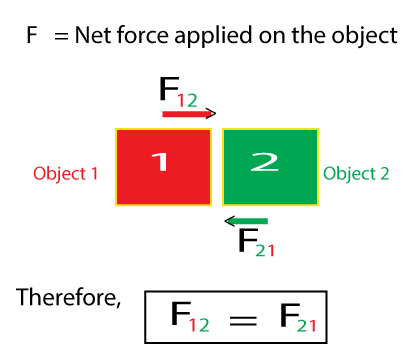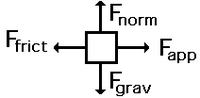# Solving Problems in Mechanics

• Difficulty Level : Easy
• Last Updated : 24 Jan, 2022

One must have probably heard of Newton’s Laws of Motion by now. These laws will assist you in addressing mechanical issues. Typically, a mechanics problem does not include numerous forces operating on a single item. On the contrary, it is concerned with an assembly of many bodies exerting forces on each other in addition to feeling gravitational pull. In this post, we will look at various mechanical problem-solving strategies.

When attempting to answer a mechanics problem, keep in mind that you may select any portion of the assembly and apply the laws of motion to that section. All you need to do is account for all forces operating on the’selected part’ as a result of the assembly’s remaining pieces. To keep things simple, we refer to the chosen component of an assembly as the’system,’ and the remaining part as the ‘environment.’

### Newton’s First Law of Motion

This law is also known as law of inertia. If the net external force on a body is zero, its acceleration is zero. Acceleration can be non-zero only if there is a net external force on the body.

Σ F = 0

dv/dt = 0

where, F is the force (summation of F means net force being applied) and v is the velocity of the object.

Applications of Newton’s First Law of Motion:

• An object is thrown in outer space moves with zero acceleration in the same direction until unless any other external object hit it with some force.
• A book lying on the table remains at rest as long as no net force acts on it.
• A marathon runner continues to run several meters beyond the finish line due to the inertia.

### Newton’s Second Law of Motion

This law is also known as law of momentum. The rate of change of momentum of a body is directly proportional to the applied force and takes place in the direction in which the force acts.

F = dp/dt

where, dp is the change in the momentum wrt change in time dt.

Applications of Newton’s Second Law of Motion:

• It is easier to push an empty cart in a supermarket than to push a loaded cart. More mass requires more power for acceleration.
• An object falling down from a certain height, undergoes an increase in acceleration because of the gravitational force applied.

### Newton’s Third Law of Motion

This law is also known as law of action and reaction. Whenever one object exerts a force on another object, the second object exerts an equal and opposite on the first.

FA = -F

F12 = F21Action-Reaction force

Applications of Newton’s Third Law of Motion:

• When we pull an elastic band, it automatically returns to its original position. The action (applied force) is stored as energy and is released as a reaction with an equal and opposite force.
• When a rocket is fired, the force of the burning gases coming out (action) exerts an equal and opposite force on the rocket (reaction) and it moves upward.

### Inertia

In practical, the particle does not change its state of rest or of uniform motion along a straight line unless it is forced to do this. This tendency of of particle to do not change its state of rest or state of uniform motion along a straight line, unless that state is changed by an external force is called as inertia.

Mass is that quantity that is solely dependent upon the inertia of an object. The more inertia that an object has, the more mass that it has. Larger the mass of the particle, smaller will be the acceleration and hence larger will be the inertia.

### Friction

The property which opposes the relative motion of the body over the surface of another body is called friction.

F = μ N

where μ is the coefficient of friction and N is the normal force.

e.g.

1. While walking friction between the ground and shoes prevent us from slipping.
2. Without friction, motion cannot be covered by belts from motor to machine.

Before going through any problem-related newton’s law of motion. You must have a strong hand over all the concepts related to it. Physics is a subject that helps us to understand the world. You should learn physics as you are helping yourself to understand how the different phenomenons happening in the world. The most inner core secret of Newton’s law of motion is the Free Body Diagram (FBD), this may help you to solve the problems very easily.Example of free body diagram (FBD)

### Sample Questions

Question 1: A passenger who is on a phone call while sitting on a train that is going at speed of 100 km/hr accidentally drops down his phone from the window. Neglecting air friction, what is the horizontal speed of the mobile phone just before it hits the ground?

According to Newton’s first law of motion, object in a motion tends to stay in a motion unless until any external force is not acting. As there is no air friction acting on a object (mobile phone) to slow down the object in the horizontal direction after it drops from the train and acceleration due to gravity would only affect in the vertical direction. So, horizontal speed of the mobile phone just before hitting ground would be approximately 100 km/hr.

Question 2: What net force is required to keep a 1.5 kg ball moving with a constant velocity of 40 m/s?

According to Newton’s first law of motion,every body continues to be in its state of rest or of uniform motion in a straight line until unless any external force is not acting.

If the net external force on a body is zero, its acceleration is zero.Hence force needed is also zero. Therefore 0 N net force required to to keep ball moving with constant velocity of 40 m/s.

Question 3: A 2000 kg of the spaceship is moving in space with a constant velocity of 1200 m/s. What is a net force acting on the spaceship (there is no gravitational force acting on the spaceship).

Newton’s first law of motion states that object remains in a motion until unless any external force is not acting on a object.In a space there is vacuum and there is no external air resistance.Hence, spaceship will travel at constant velocity of 1200 m/s with zero acceleration.

Since,  m= mass of spaceship = 2000 kg

a= acceleration of spaceship = 0

∑F = m×a

= 2000 × 0

= 0 N

Hence, net force is acting on a spaceship is 0 N.

Question 4: What is meant by static and kinetic friction?

Resistance encountered by a body in static condition while tending to move under the action of an external force is called static friction. In static friction, the frictional force resists force that is applied to an object, and the object remains at rest until the force of static friction is overcome.It is denoted as μs.

The resistance encountered by sliding body on a surface is known as kinetic friction. Kinetic friction is denoted as μk. Kinetic friction is defined as a force that acts between moving surfaces. A body moving on the surface experiences a force in the opposite direction of its movement. The magnitude of the force will depend on the coefficient of kinetic friction between the two materials.

Question 5: If a car of mass 200 kg is moving with an acceleration of 5 m/s2, then what will be the net force of a car?

Given that,

Mass of a car = Mc = 200 kg

Acceleration of a car = ac =5 m/s2

Using formula F = Mc × a

= 200 × 5

= 1000 N

Therefore, the net Force is 1000 N.

Question 6: A batter hits back a ball straight in the direction of the bowler with a velocity of 20 m/s and the initial velocity of the ball was 12 m/s.If the mass of the ball is 0.10kg. Determine the change in momentum on the ball.

Given that,

Initial velocity of the ball = 12 m/s

Final velocity of the ball = 20 m/s

Mass of the ball = 0.10kg

Change in momentum = final momentum – initial momentum

= m×v2 – m×v1

= 0.10×20 – (-0.10×12)           (ball again is in the direction from the batsman to the bowler)

= 3.2 N.s

Therefore, the change in momentum is 3.2 N.s.

Question 7: During training, a policeman fired a bullet from his gun on a wooden block, now a bullet of mass 10 gm is moving at 400 m/s penetrates 4 cm into a wooden block before coming to rest. Assuming that the force exerted by the wooden block is uniform, find the magnitude of force?

Solution:

Given that,

Mass of the bullet = Mb = 10 gm = 0.010 kg

Penetration of bullet before coming to rest = s = 4 cm = 0.04 m.

Initial velocity of bullet = Vi =400 m/s

Final velocity of bullet = Vf = 0 m/s

Here, wooden block will exert force opposite in the direction of velocity,therefore this force causes deceleration. Hence a be the deceleration in this case (-a)

By using kinematic equation,

(Vf)2 = (Vi)2 + 2as    ——(1)

0 = (400)2 – 2 × a × 0.04

a = ( (400)2 – 0 ) / 2 × 0.04

= 160000 / 0.08

= 2000000

The force on the bullet = Mb × a

= 0.01 × 2000000

= 20000 N

Question 8: A box of mass 100 kg is placed on a floor exerting some force on the floor. Determine what force does the floor exerting on the box? ( Here g= 9.81 m/s2 ).

According to Newton’s third law motion,every action there is equal and opposite reaction.Hence the force exerted by the floor on the box will be the weight of box.

Given that,

Mass of box = M = 100 kg.

weight of the box = M × g

= 100 × 9.81

= 981 N

The force exerted by the floor on the box = -981 N

This Negative sign indicates that force applied by floor is in opposite direction of force applied by the box.

Therefore, the Force applied by the floor is equal to 981 N.

Question 9: Define inertia of rest, motion, and direction?

A characteristic of matter that allows it to remain in its current condition of rest or uniform motion in a straight line until it is disrupted by an external force is called an inertia.

1. Inertia of rest: The inability of a body to change its state of rest by itself is called inertia of rest
2. Inertia of motion: The inability of a body to change its state of motion by itself is inertia of motion.
3. Inertia of direction: The inability of a body to change its direction of motion by itself inertia of direction.

Question 10: There are two passengers in an elevator who have masses that exert a force of 180 N in the downward direction. They experience a normal force upwards from the elevator floor of 207 N.At what rate they are accelerating in the upward direction? (Here g=10 m/s2)

Given that,

Upward force = 207 N

Downward force = 180 N

As they are accelerating in the upward direction then the net force-

Net Force = ∑F = Upward force – Downward force

= 207 -180

= 27 N

To find total mass of the passengers, use the equation for force of gravity,

F = m×g

m = 180/10

m = 18 Kg

To find net acceleration, use Newton’s second law of motion,

F = m × a

a = 27/18

a = 1.5 m/s2

Therefore, they are accelerating in the upward direction at the rate of 1.5m/s2.

My Personal Notes arrow_drop_up